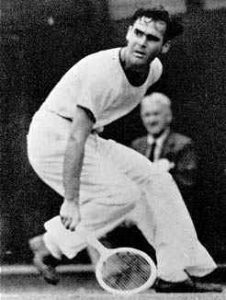Gilbert Agnew Hunt, Jr. (1916 – 2008) Mathematician and tennis player. Famous notably for his work connecting potential theory and Markov processes.

This post is devoted to a reformulation of the equilibrium measure problem of potential theory into an obstacle problem for minimal surfaces, advertised by Sylvia Serfaty.

Riesz kernel. On $\mathbb{R}^d$, for $-2 < s < d$, $$K_s=\frac{1}{s\left|\cdot\right|^s} \quad\text{with the convention}\quad K_0=-\log\left|\cdot\right|.$$ The special case $K_{d-2}$ is the Newton or Coulomb kernel. The lower bound $-2 < s$ ensures the strict convexity of an energy functional (see below) while the upper bound $s< d$ ensures integrability of the kernel and well definiteness of the potential $K_s*\mu$ of natural measures.

Riesz energy. The Riesz energy of a probability measure $\mu$ on $\mathbb{R}^d$ is
$$\mathcal{E}_s(\mu)=\iint K_s(x-y)\mu(\mathrm{d}x)\mu(\mathrm{d}y).$$ The Riesz energy with external field $V:\mathbb{R}^d\to\mathbb{R}$ (taken continuous) is
$$\mathcal{E}^V_s=\iint(K_s(x-y)+V(x)+V(y))\mu(\mathrm{d}x)\mu(\mathrm{d}y) =\mathcal{E}_s(\mu)+2\int V\mathrm{d}\mu.$$ This functional is strictly convex and has compact sub-level sets with respect to the topology of weak convergence of probability measures, in particular it is lower semi-continuous.

Equilibrium measure and Frostman conditions. The equilibrium measure $\mu_{\mathrm{eq}}$ is
$$\mu_{\mathrm{eq}}=\arg\min_\mu\mathcal{E}^V_s(\mu).$$ It depends on $d,s,V$. Since $\mathcal{E}^V_s$ is a nice quadratic form, its minimizer is characterized by nice Euler-Lagrange equations, which are known in this context as Frostman conditions. Namely $\mu_{\mathrm{eq}}$ is the unique probability measure $\mu$ for which there exists a constant $c$ such that
$$K_s*\mu+V \begin{cases} =c&\text{on \mathrm{supp}(\mu)}\\ \geq c&\text{everywhere} \end{cases}.$$

Fundamental solution. There exists a positive linear operator $L_s$ such that
$$L_s K_s=\delta_0,\quad K_s=(L_s)^{-1}.$$ More generally, for a probability measure $\mu$ on $\mathbb{R}^d$, the Riesz potential $U:=K_s*\mu$ satisfies
$$L_s U=\mu.$$ The operator $L_s$ is essentially equal to a (possibly fractional) power of the Laplacian
$$L_s=c_{s,d}(-\Delta)^{\frac{d-s}{2}}, \quad\text{in particular}\quad L_{d-2}=-c_d\Delta.$$ The Coulomb case $s=d-2$ is the unique value of $s$ for which the operator $L_s$ is local namely $L_sf(x)$ depends on $f$ locally at $x$ only. In this case we can apply $L_{d-2}$ to the Frostman variational characterization of $\mu_{\mathrm{eq}}$ to get that in the sense of distributions,
$$\mu_{\mathrm{eq}}=\frac{\Delta V}{c_d} \quad\text{on the interior of }\mathrm{supp}(\mu_{\mathrm{eq}})$$ (in particular $\mu_{\mathrm{eq}}$ has no support on $\{\Delta V<0\}$). When $s=d-2$ and $V=\left|\cdot\right|^2$ then this can be used to prove that $\mu_{\mathrm{eq}}$ is the uniform probability measure on the unit ball. Beyond the Coulomb case, the Riesz kernel is no longer local, and the computation of the equilibrium measure becomes more difficult.

Obstacle problem. Let us express the Frostman conditions in terms of the Riesz potential $U=K_s*\mu$, the operator $L_s$, and the function $\varphi=c-V$ :
$$\begin{cases} U & \geq\varphi\\ L_s U & \geq0,\text{ and =0 on \{U>\varphi\}} \end{cases}$$ in other words we have the “dichotomy”
$$\min(U-(c-V),L_s U)=0.$$ This is called an obstacle problem, where $U$ is modelling the height of a membrane and $\varphi$ an obstacle. Since $\mathrm{supp}(\mu)$ is unknown, it is a free boundary problem. The analysis of such problems in terms of existence, uniqueness, and regularity is still an active subject of research. It has also a probabilistic side, explored notably by Getoor, Chung, and others, related to first and last passage times of Lévy processes. The obstacle problem offers an alternative way to compute equilibrium measures. Last but not least, the obstacle problem admits a variational formulation with a Dirichlet energy, which is actually the convex dual problem of the equilibrium measure problem, and the probabilistic side corresponds in a way to a probabilistic interpretation of the modified Robin problem versus the Dirichlet problem.

About the links between potential theory and Markov processes, let us recall, following Hunt, that the potential kernel associated to a Markov semigroup ${(P_t)}_{t\geq0}$ is $$\int_0^\infty P_s\mathrm{d}s,$$ well understood when writing $P_s=\mathrm{e}^{-sL}$ where $-L$ is the infinitesimal generator, since formally $$\int_0^\infty\mathrm{e}^{-sL}\mathrm{d}s=L^{-1}\circ(\mathrm{id}-\pi_{\ker L}).$$